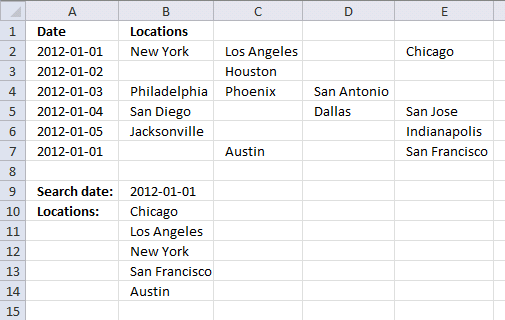Author: Oscar Cronquist Article last updated on August 17, 2017

I think I can use this to help me populate a calendar.I substituted dates for Pen, Paper, and Eraser. I then had locations substituted for \$ values.Where I have a date of say, 11/27/12, I have 10 locations delivering that day. Using the template as shown in the screen shot under "Retun multiple values horizontally or vertically (vba)" I cannot expand past column "C" to return multiple values.I think it is in the array code but I cannot figure out how to return values past column C.
If you can help, greatly appreciated!
Thanks,
JimArray formula in cell B10:

=IFERROR(INDEX(\$B\$2:\$E\$7, SMALL(IF((\$A\$2:\$A\$7=\$B\$9)*(\$B\$2:\$E\$7<>""), MATCH(ROW(\$B\$2:\$E\$7), ROW(\$B\$2:\$E\$7)), ""), ROW(A1)), 1/(SMALL(IF((\$A\$2:\$A\$7=\$B\$9)*(\$B\$2:\$E\$7<>""), MATCH(ROW(\$B\$2:\$E\$7), ROW(\$B\$2:\$E\$7))+1/MATCH(COLUMN(\$B\$2:\$E\$7), COLUMN(\$B\$2:\$E\$7)), ""), ROW(A1))-SMALL(IF((\$A\$2:\$A\$7=\$B\$9)*(\$B\$2:\$E\$7<>""), MATCH(ROW(\$B\$2:\$E\$7), ROW(\$B\$2:\$E\$7)), ""), ROW(A1)))), "")

Recommended article

Vlookup a cell range and return multiple values

VLOOKUP a multi-column range and return multiple values.

How to create an array formula

1. Select cell B10
2. Click in formula bar
3. Paste above array formula
4. Press and hold CTL + SHIFT
5. Press Enter

How to enter an array formula

Array formulas allows you to do advanced calculations not possible with regular formulas.

How to copy formula

1. Select cell B10
2. Copy cell (Ctrl + c)
3. Select cell range B11:B15
4. Paste (Ctrl + v)

### Explaining array formula in cell B10

The index function returns a value or a reference of the cell at the intersection of a particular column and row, in a given range.

INDEX(\$B\$2:\$E\$7, row_num, column_num)

The first following three steps calculate the row_nums and the remaining steps calculate column_nums.

Calculate row_nums

Step 1 - Find matching dates and non blanks

(\$A\$2:\$A\$7=\$B\$9)*(\$B\$2:\$E\$7<>"")

becomes

({40909; 40910; 40911; 40912; 40913; 40909}=40909)*({"New York","Los Angeles",0,"Chicago"; 0,"Houston",0,0; "Philadelphia","Phoenix","San Antonio",0; "San Diego",0,"Dallas","San Jose"; "Jacksonville",0,0,"Indianapolis"; 0,"Austin",0,"San Francisco"}<>"")

and returns

{1, 1, 0, 1;0, 0, 0, 0;0, 0, 0, 0;0, 0, 0, 0;0, 0, 0, 0;0, 1, 0, 1}

Step 2 - Return corresponding row numbers

IF((\$A\$2:\$A\$7=\$B\$9)*(\$B\$2:\$E\$7<>""), MATCH(ROW(\$B\$2:\$E\$7), ROW(\$B\$2:\$E\$7)), "")

becomes

IF({1, 1, 0, 1;0, 0, 0, 0;0, 0, 0, 0;0, 0, 0, 0;0, 0, 0, 0;0, 1, 0, 1}, {1;2;3;4;5;6}, "")

and returns

{1, 1, "", 1;"", "", "", "";"", "", "", "";"", "", "", "";"", "", "", "";"", 6, "", 6}

How to use the IF function

Checks if a logical expression is met. Returns a specific value if TRUE and another specific value if FALSE.

Step 3 - Return the k-th smallest value

SMALL(array, k)

SMALL(IF((\$A\$2:\$A\$7=\$B\$9)*(\$B\$2:\$E\$7<>""), MATCH(ROW(\$B\$2:\$E\$7), ROW(\$B\$2:\$E\$7)), ""), ROW(A1))

becomes

SMALL({1, 1, "", 1;"", "", "", "";"", "", "", "";"", "", "", "";"", "", "", "";"", 6, "", 6}, 1)

and returns 1.

How to use the SMALL function

The SMALL function lets you extract a number in a cell range based on how small it is compared to the other numbers in the group.

Calculate column_nums

Step 1 - Find matching dates and non blanks

(\$A\$2:\$A\$7=\$B\$9)*(\$B\$2:\$E\$7<>"")

becomes

({40909; 40910; 40911; 40912; 40913; 40909}=40909)*({"New York","Los Angeles",0,"Chicago"; 0,"Houston",0,0; "Philadelphia","Phoenix","San Antonio",0; "San Diego",0,"Dallas","San Jose"; "Jacksonville",0,0,"Indianapolis"; 0,"Austin",0,"San Francisco"}<>"")

and returns

{1, 1, 0, 1;0, 0, 0, 0;0, 0, 0, 0;0, 0, 0, 0;0, 0, 0, 0;0, 1, 0, 1}

Step 2 - Calculate both row numbers and column numbers

IF((\$A\$2:\$A\$7=\$B\$9)*(\$B\$2:\$E\$7<>""), MATCH(ROW(\$B\$2:\$E\$7), ROW(\$B\$2:\$E\$7))+1/MATCH(COLUMN(\$B\$2:\$E\$7), COLUMN(\$B\$2:\$E\$7)), "")

becomes

IF({1, 1, 0, 1;0, 0, 0, 0;0, 0, 0, 0;0, 0, 0, 0;0, 0, 0, 0;0, 1, 0, 1}, {1;2;3;4;5;6}+{1,0.5,0.333333333333333,0.25}, "")

and returns

{2,1.5,"",1.25;"","","","";"","","","";"","","","";"","","","";"",6.5,"",6.25}

Step 3 - Return the k-th smallest value

SMALL(array, k)

SMALL(IF((\$A\$2:\$A\$7=\$B\$9)*(\$B\$2:\$E\$7<>""), MATCH(ROW(\$B\$2:\$E\$7), ROW(\$B\$2:\$E\$7))+1/MATCH(COLUMN(\$B\$2:\$E\$7), COLUMN(\$B\$2:\$E\$7)), ""), ROW(A1))

becomes

SMALL({2,1.5,"",1.25;"","","","";"","","","";"","","","";"","","","";"",6.5,"",6.25}, 1)

and returns 1.25.

Step 4 - Subtract row numbers

SMALL(IF((\$A\$2:\$A\$7=\$B\$9)*(\$B\$2:\$E\$7<>""), MATCH(ROW(\$B\$2:\$E\$7), ROW(\$B\$2:\$E\$7))+1/MATCH(COLUMN(\$B\$2:\$E\$7), COLUMN(\$B\$2:\$E\$7)), ""), ROW(A1))-SMALL(IF((\$A\$2:\$A\$7=\$B\$9)*(\$B\$2:\$E\$7<>""), MATCH(ROW(\$B\$2:\$E\$7), ROW(\$B\$2:\$E\$7)), ""), ROW(A1))

becomes

SMALL({2,1.5,"",1.25;"","","","";"","","","";"","","","";"","","","";"",6.5,"",6.25}, 1)-SMALL({1, 1, "", 1;"", "", "", "";"", "", "", "";"", "", "", "";"", "", "", "";"", 6, "", 6}, ROW(A1))

becomes

1.25-1

and returns 0.25

Step 5 - Calculate column number

1/(SMALL(IF((\$A\$2:\$A\$7=\$B\$9)*(\$B\$2:\$E\$7<>""), MATCH(ROW(\$B\$2:\$E\$7), ROW(\$B\$2:\$E\$7))+1/MATCH(COLUMN(\$B\$2:\$E\$7), COLUMN(\$B\$2:\$E\$7)), ""), ROW(A1))-SMALL(IF((\$A\$2:\$A\$7=\$B\$9)*(\$B\$2:\$E\$7<>""), MATCH(ROW(\$B\$2:\$E\$7), ROW(\$B\$2:\$E\$7)), ""), ROW(A1)))

becomes

1/0.25 and returns column number 4.